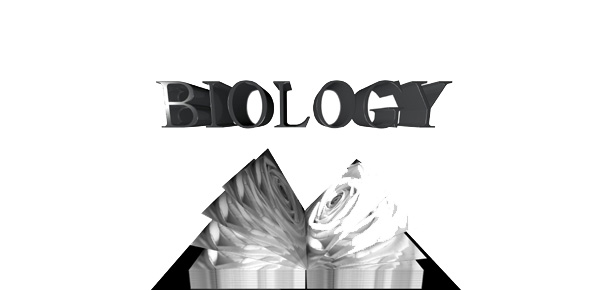# AP Bio Population Quiz

12 Questions | Attempts: 91Settings• 1.
When the size of a population greatly exceeds K, it would be expected the population to
• A.

Rapidly increase

• B.

Slowly increase.

• C.

Slowly decrease

• D.

Rapidly decrease.

• E.

Stabilize

• 2.
The logistic equation:
• A.

Models a J-shaped growth curve.

• B.

Takes into account the carrying capacity of the environment.

• C.

Predicts continued exponential growth.

• D.

Would characterize the uncontrolled growth of a population.

• E.

Models a K-shaped growth curve.

• 3.
When r = 0, the population size:
• A.

Will increase

• B.

Will decrease

• C.

Is stable

• D.

Depends upon population size

• E.

Depends on carrying capacity

• 4.
In models of logistic population growth,
• A.

Population growth rate slows dramatically as N approaches K.

• B.

New individuals are added to the population most rapidly at the beginning of the population's growth.

• C.

Only density-dependent factors affect the rate of population growth

• D.

Only density-independent factors affect the rate of population growth

• E.

Carrying capacity is never reached.

• 5.
Which of the following characterizes relatively K-selected populations?
• A.

Offspring with good chances of survival

• B.

Many offspring per reproductive episode

• C.

Small offspring

• D.

A high intrinsic rate of increase

• E.

Early parental reproduction

• 6.
Carrying capacity is
• A.

Seldom reached by marine producers and consumers because of the vast resources of the ocean.

• B.

The maximum population size that a particular environment can support.

• C.

Fixed for most species and unchanging, even when environments change.

• D.

Determined by density and dispersion data.

• E.

The term used to describe the stress a population undergoes due to limited resources.

• F.

The term used to describe the stress a population undergoes due to limited resources.

• 7.
Generally, r-selected organisms exhibit:
• A.

Short life spans and early maturity.

• B.

Long life spans and extended parental care of offspring.

• C.

Long development time to maturity, but small body size.

• D.

Large body size and extended parental care of offspring.

• E.

Large body size, but short life spans.

• 8.
A caribou dies because it is weakened by parasites, such as tapeworms, passed from one individual to the next under crowded conditions.  What killed the caribou?
• A.

Density-independent factors

• B.

Density-dependent factors

• C.

Abiotic factors

• D.

Biotic factors

• E.

Density-dependent and biotic factors

• F.

Density-independent and abiotic factors

• 9.
Human population is currently following a J-shaped curve.  This means that the population is growing
• A.

Arithmetically

• B.

Sigmoidally

• C.

At a decreasing rate

• D.

At carrying capacity

• E.

Exponentially

• 10.
A population will grow exponentially when
• A.

The birth rate and death rate are equal

• B.

The birth rate exceeds the death rate and there is no immigration or emigration

• C.

Emmigration exceeds immigration and the birth rate equals the death rate

• D.

The birth rate equals the death rate and immigration is equal to emigration

• E.

The carrying capacity is exceeded

• 11.
All of the individuals of a single species within a community is a(n)
• A.

Ecosystem

• B.

Population

• C.

Biome

• D.

Community

• E.

Niche

• 12.
Which of the following species are capable of exhibiting exponential growth?
• A.

Many bacteria

• B.

Fruit flies

• C.

Humans

• D.

Bald Eagles

• E.

All speciesBack to top
×

Wait!
Here's an interesting quiz for you.